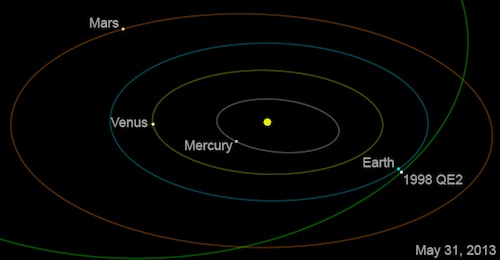## Plus Blog

August 1, 2013

This year's British Science Festival will take place from 7 - 12 September at the University of Newcastle and there's plenty of (mostly free) maths on offer.

You can take part in some pioneering maths at PrimeGrid: Search for a world record prime number on 7 September. Learn about prime numbers with more than a thousand digits, the history of the search for large primes, and how you can use your computer to join PrimeGrid and find new primes of your own.

The randomness show on 8 September explores randomness in your life, and the ways in which maths can order the chaos around us. You can delve into mathematical history, discover how maths can be used to predict the future and uncover the hidden maths inside you.

Be a maths millionaire on 11 September is all about famous mathematical equations and the adventures of the mathematicians working on them, including those that make Google work.

If you're interested in economics then An insight into financial markets and trading on 11 September may be for you: it will have a real time interactive workshop exploring financial markets. You can get to grips with data, charts and financial information in the trading room. This event costs £4.

Slide into the maths museum on 7 September explores the maths needed to crack the fastest slide and build skateboarding tracks. The UK's first museum entirely dedicated to mathematics wants to know what you would like at the museum. This event costs £10.85 for adults and there are various concession rates.

For the beady-eyed, see if you can spot Dr Math's giant logic puzzles all around the festival and how many of his mind-bending brain-twisters you can solve.

Space for some events is limited, so book now to reserve your place at www.britishsciencefestival.org or call 08456 807 207 for more information.

July 16, 2013

Number theory is famous for problems that everyone can understand and that are easy to express, but that are fiendishly difficult to prove. Here are some of our favourites.

### The Goldbach conjecture

The Goldbach conjecture is named after the mathematician Christian Goldbach who formulated it in the middle of the eighteenth century. It states that any even natural number greater than 2 can be written as the sum of two prime numbers.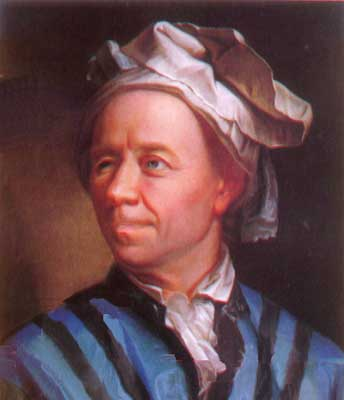Leonard Euler (1707-1783) corresponded with Christian Goldbach about the conjecture now named after the latter.

It is easy to see that this is true for the first few even numbers greater than 2:

4=2+2
6=3+3
8=3+5
10=5+5=3+7.

This seems so straightforward you might be tempted to try and prove it yourself — and you'd be in very good company as some of the brightest mathematical minds have been chiselling away at the conjecture ever since it was first pronounced. But so far without success. The closest result that has been proved, in 1995, says that every even number is the sum of at most six primes.

There is a similar statement, called the weak Goldbach conjecture, which says that every odd natural number greater than 5 is the sum of three primes. Again we can see that this is true for the first few odd numbers greater than 5:

7 = 3+2+2
11=3+3+5
13=3+5+5
17=5+5+7.

This statement is called "weak" because once someone finds a proof for the ordinary "strong" Goldbach conjecture, the weak one can be deduced from it.

In 1938 Nils Pipping showed that the (strong) Goldbach conjecture is true for even numbers up to and including 105. The latest result, established using a computer search, shows it is true for even numbers up to and including 4 x 1018 — that's a huge number, but for mathematicians it isn't good enough. Only a general proof will do.

### Perfect numbers

A perfect number is a number that's the sum of all of its divisors (excluding itself). For example, 6 is a perfect number because its divisors (apart from 6 itself) are 1, 2 and 3, and

6 = 1 + 2 + 3.

The next perfect number is 28, which has divisors 1, 2, 4, 7 and 14, and:

28 = 1 + 2 + 4 + 7 + 14.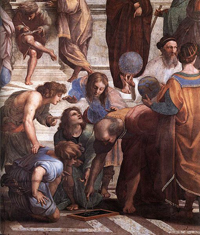Euclid, depicted with a compass in Raphael's painting The school of Athens.

The next three perfect numbers are 496, 8128 and 33,550,336.

The gaps between perfect numbers are as wide as their discovery has been painstaking. The first four perfect numbers seem to have been known to the Greeks, the fifth and sixth weren't written down explicitly until the 15th century and the seventh followed in the 16th century. Today we know of 48 perfect number, the largest of which has over 34 million digits. All of these 48 are even. This raises two questions:

• Are there infinitely many perfect numbers?
• Are there any odd perfect numbers?

So far no one has been able to answer these questions with a conclusive proof.

One thing that was already known to the Greek mathematician Euclid over 2,000 years ago is that if p is a prime number and 2p-1 is also a prime number, then 2p-1(2p-1) is an even perfect number. For example,

21(22-1) = 6
22(23-1) = 28.

The 18th century mathematician Leonhard Euler proved that every even perfect number is of this form. The largest known perfect number, the one with over 34 million digits, is

257885160 x (257885161−1).

This leads us straight to our next number mystery.

### Mersenne primes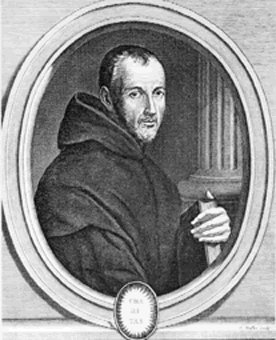Marin Mersenne, (1588-1648).

Prime numbers are those numbers that are divisible only by themselves and 1. The first few are 2, 3, 5, 7, and 11. Unlike for perfect numbers we do know that there are infinitely many of them. The proof for that was furnished by Euclid, however there is no easy recipe that generates all the prime numbers. This is where numbers of the form 2p-1, where p is a prime, come in useful. These are called Mersenne numbers, after the French monk Marin Mersenne (1588-1648) who studied them, and they have a good chance of being prime themselves.

The question is, are there infinitely many such Mersenne primes? Mathematicians believe that there probably are, but again nobody has as yet been able to prove that conjecture. A total of 48 Mersenne primes have been found so far, the largest, discovered in January 2013, being

257885161 − 1.

These correspond to the 48 known perfect numbers. The search for larger and larger Mersenne primes continues, as does the search for a conclusive proof that there are infinitely many.

### And there is more...

Another favourite number theory mystery is the twin prime conjecture, which states that there are infinitely many pairs of primes that are 2 apart. There's been recent progress on this, so we refer you to our news story. One mystery that has been solved, after over 350 years of effort, is Fermat's last theorem. We recently celebrated the twentieth anniversary of the announcement of its proof — you can find out more here. That's probably enough to fill a minute, but if you haven't had enough you can read more about number theory, prime numbers, Mersenne primes and the search for larger and larger primes here on Plus.

July 3, 2013

Over 2000 years ago the Greek mathematician Euclid came up with a list of five postulates on which he thought geometry should be built. One of them, the fifth, was equivalent to a statement we are all familiar with: that the angles in a triangle add up to 180 degrees. However, this postulate did not seem as obvious as the other four on Euclid's list, so mathematicians attempted to deduce it from them: to show that a geometry obeying the first four postulates would necessarily obey the fifth. Their struggle continued for centuries, but in the end they failed. They found examples of geometries that do not obey the fifth postulate.

### Spherical geometry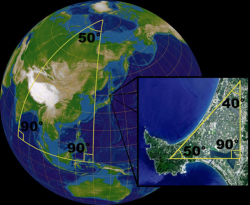Image: Lars H. Rohwedder.

Spherical geometry is geometry on a sphere. In spherical geometry the Euclidean idea of a line becomes a great circle, that is, a circle of maximum radius spanning right around the fattest part of the sphere. It is no longer true that the sum of the angles of a triangle is always 180 degrees. Very small triangles will have angles summing to only a little more than 180 degrees (because, from the perspective of a very small triangle, the surface of a sphere is nearly flat). Bigger triangles will have angles summing to very much more than 180 degrees.

One funny thing about the length of time it took to discover spherical geometry is that it is the geometry that holds on the surface of the Earth! But we never really notice, because we are so small compared to the size of the Earth that if we draw a triangle on the ground, and measure its angles, the amount by which the sum of the angles exceeds 180 degrees is so tiny that we can't detect it.

The sphere has what mathematicians call positive curvature and this makes intuitive sense. But there is another geometry that takes things in the other direction:

### Hyperbolic geometry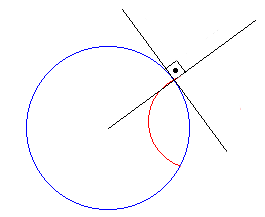Hyperbolic geometry isn't as easy to visualise as spherical geometry because it can't be modelled in three-dimensional Euclidean space without distortion. One way of visualising it is called the Poincaré disc.

Take a round disc, like the one bounded by the blue circle in the figure on the right, and imagine an ant living within it. In Euclidean geometry the shortest path between two points inside that disc is along a straight line. In hyperbolic geometry distances are measured differently so the shortest path is no longer along a Euclidean straight line but along the arc of a circle that meets the boundary of the disc at right angles, like the one shown in red in the figure. A hyperbolic ant would experience the straight-line path as a detour — it prefers to move along the arc of such a circle.

A hyperbolic triangle, whose sides are arcs of these semicircles, has angles that add up to less than 180 degrees. All the black and white shapes in the figure on the left are hyperbolic triangles.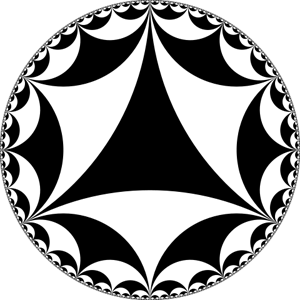One consequence of this new hyperbolic metric is that the boundary circle of the disc is infinitely far away from the point of view of the hyperbolic ant. This is because the metric distorts distances with respect to the ordinary Euclidean one. Paths that look the same length in the Euclidean metric are longer in the hyperbolic metric the closer they are to the boundary circle. The figure below shows a tiling of the hyperbolic plane by regular heptagons. Because of the distorted metric the heptagons are all of the same size in the hyperbolic metric. And as we can see the ant would need to traverse infinitely many of them to get to the boundary circle — it is infinitely far away!

In contrast to the sphere with its positive curvature, the hyperbolic plane is negatively curved. Very small regions of it have the same type of curvature as saddles: along one direction, they look like the summit of a mountain ridge, and along another direction they look like the bottom of a valley.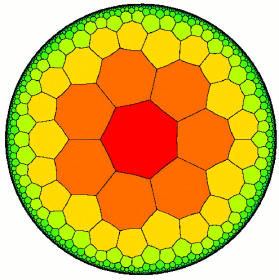Image created by David Wright.

Hyperbolic geometry may look like a fanciful mathematical construct but it has real-life uses. When Einstein developed his special theory of relativity in 1905 he found that the symmetries of hyperbolic geometry were exactly what he needed to formulate the theory. Today mathematicians believe that hyperbolic geometry may help to understand large networks like Facebook or the Internet.

You can read more about hyperbolic geometry in non-Euclidean geometry and Indra's pearls.

June 28, 2013

Right. That's it. We're convinced. We are officially behind team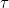! Pronounced "tau",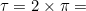6.28318... There is a movement that it should replace the use of that favourite mathematical constant,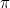. We're not sure if it's likely thatwill go gently into that dark night, but Phil Moriaty certainly makes a good case for the use ofin teaching in this episode of Numberphile, released in honour of today being Tau day (June 28 is 6.28 when written in the US date format).

And Vi Hart explains that not only isthe logical choice, it will return beauty to trigonometry...

Phew. Now we know that Euler's identity is safe in's hand, we definitely vote! Happy Tau Day everyone!

May 31, 2013Suppose you have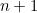people in a room and each person shakes hands with each other person once. How many handshakes do you get in total? The first person shakes hands with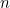other people, the second shakes hands with the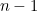remaining people, the third shakes hands with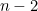remaining people, etc, giving a total of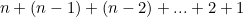handshakes.

But we can also look at this in another way: each person shakes hands withothers and there arepeople, giving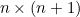handshakes. But this counts every handshake twice, so we need to divide by 2, giving a total of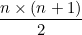handshakes.

Putting these two arguments together, we have just come up with the formula for summing the firstintegers and we’ve proved that it is correct: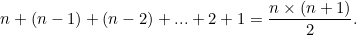Maths can be so easy!

This puzzle is inspired by content on our sister site Wild Maths, which encourages students to explore maths beyond the classroom and designed to nurture mathematical creativity. The site is aimed at 7 to 16 year-olds, but open to all. It provides games, investigations, stories and spaces to explore, where discoveries are to be made. Some have starting points, some a big question and others offer you a free space to investigate.